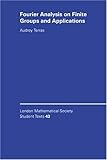## Representation Theory of Finite Groups

#### A very brief history of the subject:

While representation theory of finite abelian groups, and the corresponding character theory , goes back to the classical works of Lagrange, Gauss, and Dirichlet in early 19th century, the non-abelian representation theory and character theory started in 1890's in a series of ground breaking papers by Frobenius. These papers were written in response to a question posed by Dedekind about factorization of a certain multi-variable polynomial naturally attached to any group. New, and much more transparent, foundations for representation theory were obtained a few years later by Isai Schur (a student of Frobenius). A more algebraic approach, based on module theory of semi-simple algebras and the Artin-Wedderburn theorem, was also discovered later by Emmy Noether. Relations between representation theory and harmonic analysis (Fourier analysis) were gradually discovered so that after 1930's it was very hard to separate the two subjects in any reasonable way.

#### Outlines and Lecture notes:

Here is a brief outline of some of the topics the topics to be covered:

• ###### Abelian theory:
• Representations and characters of finite abelian groups;
• Orthogonality relations; examples: Dirichlet characters and L-functions,
• The dual of an abelian group; Pontryagin duality
• applications: discrete Fourier transform; fast Fourier transform; quantum computing (not sure to include this one), law of quadratic reciprocity
• Poisson summation formula
• ###### Non-abelian theory:
• General notions of representation theory; irreducible representations, complete reducibility, Schur's lemma, orthogonality relations,
• The unitary dual of a finite group, Fourier transform for finite groups, Pleancherel formula, main theorems.Peter-Weyl theorem. Induced represenations, Frobenius charcater formula, tensor products of represenations
• The character table
• Representations of symmetric groups.

time permiting I shall also look at corresponding results for compact topological groups through examples.

Marking Policy:  No exams. Marks will be based on take home assignments.

#### Assignments:

Assignement 1: pdf Due Date:  Mon 5/9

Assignement 2: pdf Due Date:  .....

#### Textbooks:

I shall mostly follow the following text byA. Terras, Fourier Analysis on Finite Groups and Applications (London Mathematical Society Student Texts), 19... (The word analysis in the title should not give the impression that we use analysis in any serious way in this course. In fact the main background needed to understand the course is finite dimensional linear algebra).

A couple of other interesting texts for your own self study are:S. Sternberg, Group Theory and Physics,....J. P. Serre, Linear Representations of Finite Groups,....

The online notes of Etingof should be useful too.